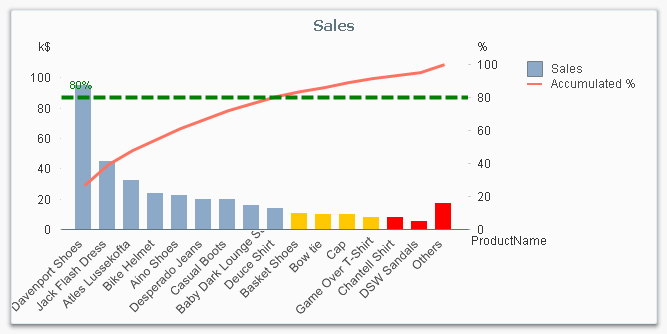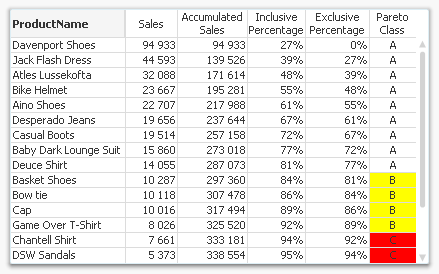# Qlik Design Blog

All about product and Qlik solutions: scripting, data modeling, visual design, extensions, best practices, etc.Employee

## Recipe for a Pareto Analysis

“Which products contribute to the first 80% of our turnover?”

This type of question is common in all types of business intelligence. I say “type of question” since it appears in many different forms: Sometimes it concerns products, but it can just as well concern customers, suppliers or sales people. It can really be any dimension. Further, here the question was about turnover, but it can just as well be number of support cases, or number of defect deliveries, etc. It can in principle be any additive measure.

It is called Pareto analysis. Sometimes also known as 80/20 analysis or ABC analysis.

The logic is that you first sort the products according to size, then accumulate the numbers, and finally calculate the accumulated measure as a percentage of the total. The products contributing to the first 80% are your best products; your “A” products. The next 10% are your “B” products, and the last 10% are your “C” products.And here’s how you do it in QlikView:

1. Create a pivot table and choose your dimension and your basic measure. In my example, I use Product and Sum(Sales).

2. Sort the chart descending by using the measure Sum(Sales) as sort expression. It is not enough just to check “Sort by Y-value”.

3. Add a second expression to calculate the accumulated sales value:
RangeSum(Above(Sum(Sales), 0, RowNo()))
Call this expression Accumulated Sales. The Above() function will return an array of values – all above values in the chart – and the RangeSum() function will sum these numbers.

4. Create a third expression from the previous one; one that calculates the accumulated sales in percent:
RangeSum(Above(Sum(Sales), 0, RowNo())) / Sum(total Sales)
Format it as a percentage and call it Inclusive Percentage.

5. Create a fourth expression from the previous one; one that calculates the accumulated sales in percent, but this time excluding the current row:
RangeSum(Above(Sum(Sales), 1, RowNo())) / Sum(total Sales)
Format it as a percentage and call it Exclusive Percentage.

6. Create a fifth expression for the ABC classification:
If([Exclusive Percentage] <= 0.8, 'A', If([Exclusive Percentage] <= 0.9, 'B', 'C'))
Call this expression Pareto Class. The reason why the Exclusive Percentage is used, is that the classification should be determined by the lower bound of a product’s segment, not the upper.

7. Create a conditional background color, e.g.
If([Pareto Class] = 'C', LightRed(), If([Pareto Class] = 'B', Yellow()))

You should now have a table similar to the following. In it you can clearly see the classification of different products.In this table, there are five different expressions that you can use for Pareto analysis. The graph in the beginning of this post uses Sales and Inclusive Percentage for the bars and the line, respectively; and Pareto Class for the coloring of the bars.

Further, you may want to combine the Pareto Class and the Exclusive Percentage into one expression:

Pareto Class =
If(RangeSum(Above(Sum(Sales),1,RowNo())) / Sum(total Sales) <= 0.8, 'A',
If(RangeSum(Above(Sum(Sales),1,RowNo())) / Sum(total Sales) <= 0.9, 'B', 'C'))

Good luck in creating your Pareto chart.

HIC

Further reading related to this topic:

Recipe for an ABC Analysis

Recipe for a Pareto Analysis – Revisited

New Contributor II

Hi Henric - this is an amazing walkthrough. Is it possible to do it for a cyclic group as the dimension?

171 Views
New Contributor II

Did you figure this out? I assume its because you had a cyclic group.

171 Views
New Contributor

How I change the order?

164 ViewsPartner

Hi Henric,

Hope you are good!

Thanks for this wonderful recipe. We have used this example multiple times in multiple ways and the response was amazing!

However, is there any way , we can enter the N% in an Input Box (variable) that would show the Top N% in the Straight Table chart?

I have posted a question in this thread too -

https://community.qlik.com/t5/QlikView-App-Development/Input-Box-N-will-show-the-Cumulative-sum-of-R...

Regards!

143 Views
New Contributor II

Good Morning All,

I am working on Customer Complaints Analysis. I have read the article, which is very helpful:

I used the same formula but instead of SUM i used COUNT

My data base contain in general:

 Case_No Complaint_Date Product_Code Complaint_Category Complaint_Type

Combination of Case_No&Complaint_Date&Product_Code is always unique and it has auto numbering ComplaintID, which I am counting

I have 9 Complaint Categories

Each Categories has at around 5 types

When I setup it accordingly with instructionMy Pareto charto doesn't work properlyHaving below setups:My Parteo Chart working fine, however sorting order for first column is not working, for some of the reasons is working for second and rest of the columns.When I change  Complaint_Cat to Complaint Type(rest setups and formulas are exactly the same), my chart doesn't work at all. Bar chart seems to be working, but Pareto Class is mixed.Could you please advise? I think, I am doing some simple mistake but I am not sure where I should look at first,

Thank you

Best Regards

Michael

70 Views